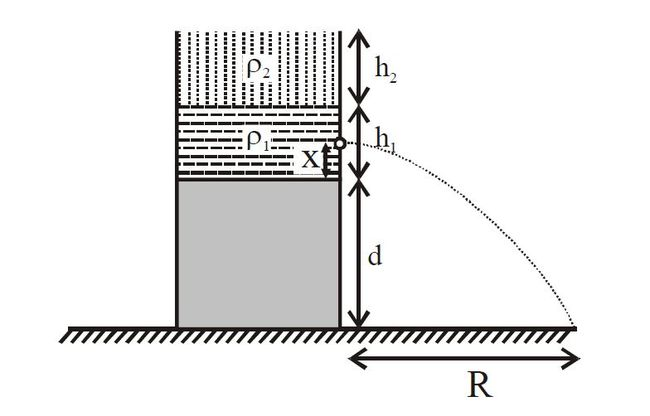# Fluids+Efflux+RangeA vessel having two immiscible fluids having density $\rho_1$ and $\rho_2$ filled upto height $h_1$ and $h_2$ placed on a block of height $d$ as shown in figure. There is a hole at a distance $x$ from the bottom of vessel. For all $x \in (0, h_1)$ if $x$ increases, range $R$ increases. Choose the correct relation.

×

Problem Loading...

Note Loading...

Set Loading...